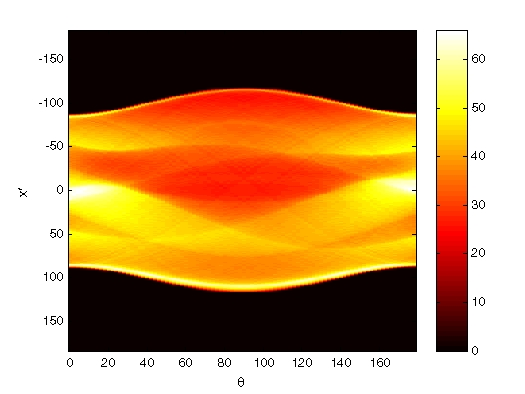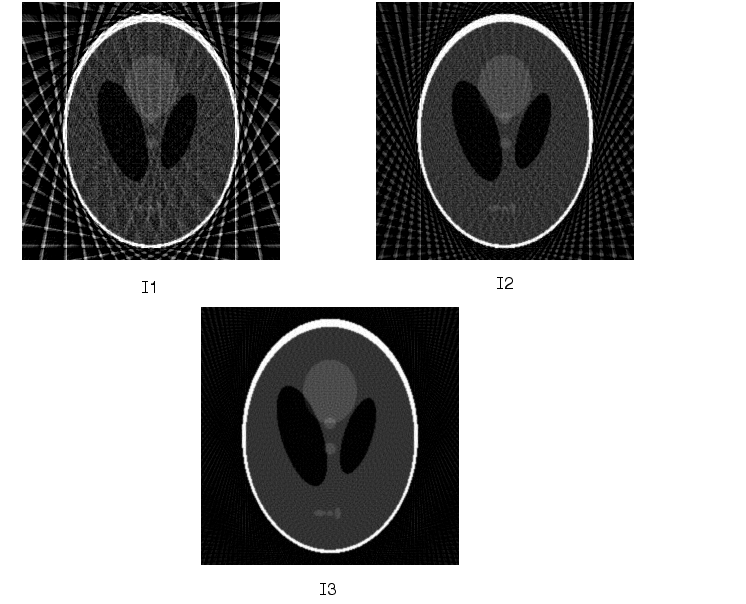Image Processing Toolbox User's GuideExample: Reconstructing an Image from Parallel Projection Data

The commands below illustrate how to reconstruct an image from parallel projection data. The test image is the Shepp-Logan head phantom, which can be generated by the Image Processing Toolbox function phantom. The phantom image illustrates many of the qualities that are found in real-world tomographic imaging of human heads. The bright elliptical shell along the exterior is analogous to a skull, and the many ellipses inside are analogous to brain features.

1. Create a Shepp-Logan head phantom image.
• P = phantom(256);
imshow(P)2. Compute the Radon transform of the phantom brain for three different sets of theta values. R1 has 18 projections, R2 has 36 projections, and R3 has 90 projections.
• theta1 = 0:10:170; [R1,xp] = radon(P,theta1);
theta2 = 0:5:175;  [R2,xp] = radon(P,theta2);
theta3 = 0:2:178;  [R3,xp] = radon(P,theta3);

3. Display a plot of one of the Radon transforms of the Shepp-Logan head phantom. The following figure shows R3, the transform with 90 projections.
• figure, imagesc(theta3,xp,R3); colormap(hot); colorbar
xlabel('\theta'); ylabel('x\prime');1. Note how some of the features of the input image appear in this image of the transform. The first column in the Radon transform corresponds to a projection at 0º that is integrating in the vertical direction. The centermost column corresponds to a projection at 90º, which is integrating in the horizontal direction. The projection at 90º has a wider profile than the projection at 0º due to the larger vertical semiaxis of the outermost ellipse of the phantom.

1. Reconstruct the head phantom image from the projection data created in step 2 and display the results.
• I1 = iradon(R1,10);
imshow(I1)
figure, imshow(I2)
figure, imshow(I3)

1. The following figure shows the results of all three reconstructions. Notice how image I1, which was reconstructed from only 18 projections, is the least accurate reconstruction. Image I2, which was reconstructed from 36 projections, is better, but it is still not clear enough to discern clearly the small ellipses in the lower portion of the image. I3, reconstructed using 90 projections, most closely resembles the original image. Notice that when the number of projections is relatively small (as in I1 and I2), the reconstruction can include some artifacts from the back projection.Inverse Radon Transform Fan-Beam Projection Data